Transfer characteristic [output voltage $\left({V}_{0}\right)$ vs input voltage $\left({V}_{i}\right)$] for a base biased transistor in CE configuration is as shown in the figure.For using transistor as a switch, it is used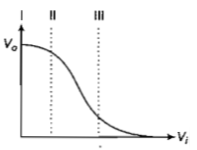(a) in region III

(b) both in region (I) and (III)

(c) in region II

(d) in region I

Concept Questions :-

Transistor
High Yielding Test Series + Question Bank - NEET 2020

Difficulty Level:

The figure shows a logic circuit with two inputs A and B and the output C.The voltage wave forms across A, B and C are as given.The logic circuit gate is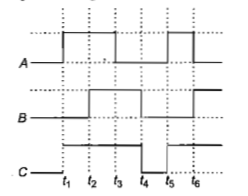1. OR gate                                          2. NOR gate

3. AND gate                                        4. NAND gate

Concept Questions :-

Logic gates
High Yielding Test Series + Question Bank - NEET 2020

Difficulty Level:

The input resistance of a silicon transistor is

100 $\mathrm{\Omega }$. Base current is changed by 40 $\mathrm{\mu A}$

which results in a change in collector current

by 2 mA. This transistor is used as a common-

emitter amplifier with a load resistance of 4 k$\mathrm{\Omega }$.

The voltage gain of the amplifier is

1. 2000

2. 3000

3. 4000

4. 1000

Concept Questions :-

Transistor
High Yielding Test Series + Question Bank - NEET 2020

Difficulty Level:

To get an output Y=1 in given circuit, which

of the following input will be correct ?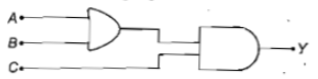A     B      C                   A     B     C

1.  1     0       0             2.  1     0     1

3. 1      1       0             4.  0     1     0

Concept Questions :-

Logic gates
High Yielding Test Series + Question Bank - NEET 2020

Difficulty Level:

Symbolic representation of four logic gates

are shown as

(i)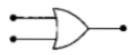(ii)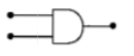(iii)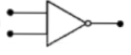(iv)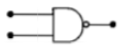Pick out which ones are for AND, NAND and

NOT gates, respectively.

(a) (iii), (ii)and (i)

(b) (iii), (ii) and (iv)

(c) (ii), (iv) and (iii)

(d) (ii), (iii) and (iv)

Concept Questions :-

Logic gates
High Yielding Test Series + Question Bank - NEET 2020

Difficulty Level:

If a small amount of antimony is added to germanium crystal

(a) the antimony becomes an acceptor atom

(b) there will be more free electrons than holes in the semiconductor

(c) its resistance is increased

(d) it becomes a p-type semiconductor

Concept Questions :-

Types of semiconductors
High Yielding Test Series + Question Bank - NEET 2020

Difficulty Level:

In forward biasing of the p-n junction

(a) the positive terminal of the battery is connected to n-side and the depletion region becomes thin

(b) the positive terminal of the battery is connected to n-side and the depletion region becomes thick

(c) the positive terminal of the battery is connected to p-side and the depletion region becomes thin

(d) the positive terminal of the battery is connected to p-side and the depletion region becomes thick

Concept Questions :-

PN junction
High Yielding Test Series + Question Bank - NEET 2020

Difficulty Level:

A transistor is operated in common emitter configuration at  such that a change in the base current from  to  produces a change in the collector current from  to . The current gain is

1. 75                                               2. 100

3. 25                                                4. 50

Concept Questions :-

Transistor
High Yielding Test Series + Question Bank - NEET 2020

Difficulty Level:

In the following figure, the diodes which are forward biased, are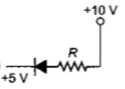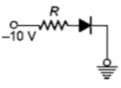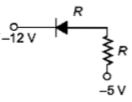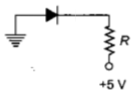(1) C and D only

(2) A and C only

(3) B only

(4) Band D only

Concept Questions :-

PN junction
High Yielding Test Series + Question Bank - NEET 2020

Difficulty Level:

Pure Si at 500 K has equal number of electron $\left({n}_{\mathit{e}}\right)$ and hole $\left({n}_{\mathit{h}}\right)$ concentrations of $1.5×{10}^{16}{\mathrm{m}}^{-3}.$ Doping by indium increases ${n}_{\mathit{h}}$ to  The doped semiconductor is of

(a) n-type with electron concentration

(b) p-type with electron concentration

(c) n-type with electron concentration

(d) p-type with electron concentration

Concept Questions :-

Types of semiconductors